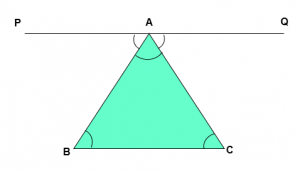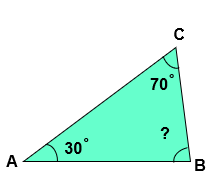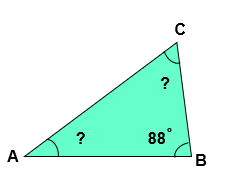# Angle Sum Property of a Triangle

Proof of Angle Sum Property of a Triangle

Theorem – The sum of the angles of a triangle is 180˚Proof – Consider a ∆ ABC

Draw a line PQ parallel to BC

∠PAB + ∠BAC + ∠ CAQ = 180˚ [Angle on straight line is equal to 180˚ ] —  1

Now BC || PQ and transversal AB cuts BC at B and PQ at A

∠PAB = ∠ABC   [Alternate angles ]  —  2

BC || PQ and transversal AC cuts BC at C and PQ at A

∠CAQ = ∠ACB    [Alternate angles]  — 3

From Equation 1, 2 and 3 we get,

∠ABC  + ∠BAC + ∠ACB  = 180˚

Hence, the sum of the angles of a triangle is 180˚.

## Angle Sum Property of a Triangle Examples –

EXAMPLE 1

In the given triangle ABC,  ∠BAC = 30˚, ∠ACB = 70˚, find the measure of ∠ABC ?EXPLANATION

Given:

∠BAC = 30˚

∠ACB = 70˚

∠ABC = ?

According to Angle Sum Property of a triangle

∠BAC + ∠ACB +∠ABC = 180˚

30˚ + 70˚ + ∠ABC = 180˚

100˚ + ∠ABC = 180˚

∠ABC = 180˚ – 100˚

∠ABC = 80˚

Hence, the measure of ∠ABC is 80˚

EXAMPLE 2

In the given triangle ABC,  ∠ABC = 88 and ∠ACB = ∠BAC , then find the measure of ∠ACB and ∠BAC ?EXPLANATION

Given:

∠ABC = 88˚

∠ACB = ?

∠BAC = ?

Let ∠ACB = ∠BAC = a

According to Angle Sum Property of a triangle

∠ABC + ∠ACB +∠BAC = 180˚

88˚ + a + a = 180˚

88˚ + 2a = 180˚

2a = 180˚ –  88˚

a = 92⁄2

a = 46˚

Hence, ∠ACB = ∠BAC = a = 46˚

EXAMPLE 3

Find the angles of a triangle which are in the ratio 7 : 2 : 3

EXPLANATION

Let the measures of the given angles be:

1st angle = ( 7a )˚

2nd angle = ( 2a )˚

3rd angle = ( 3a )˚

Then, According to angle sum property of a triangle

1st angle + 2nd angle + 3rd angle = 180 ˚

7a + 2a + 3a = 180

12a = 180

a = 180⁄12

a = 15

So, 1st angle = ( 7a )˚ = ( 7 x a )˚ = ( 7 x 15 )˚ = 105˚

2nd angle = ( 2a )˚ = ( 2 x a )˚ = ( 2 x 15 )˚ = 30˚

3rd angle = ( 3a )˚ = ( 3 x a )˚ = ( 3 x 15 )˚ = 45˚

Hence, the measures of the angles of the triangle are 105˚ , 30˚ and 45˚